# Simplifying Expressions Worksheet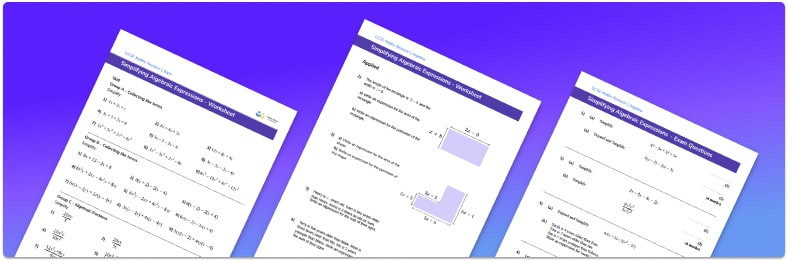Help your students prepare for their Maths GCSE with this free simplifying expressions worksheet of 35 questions and answers

• Section 1 of the simplifying expressions worksheet contains 27 skills-based simplifying expressions questions, in 3 groups to support differentiation
• Section 2 contains 4 applied simplifying expressions questions with a mix of worded problems and deeper problem solving questions
• Section 3 contains 4 foundation and higher level GCSE exam style simplifying expressions questions
• Answers and a mark scheme for all simplifying expressions questions are provided
• Questions follow variation theory with plenty of opportunities for students to work independently at their own level
• All questions created by fully qualified expert secondary maths teachers
• Suitable for GCSE maths revision for AQA, OCR and Edexcel exam boards
• To receive this resource and regular emails with more free resources, blog posts and other Third Space updates, enter your email address and click below.

• This field is for validation purposes and should be left unchanged.

You can unsubscribe at any time (each email we send will contain an easy way to unsubscribe). To find out more about how we use your data, see our privacy policy.

### Simplifying expressions at a glance

Simplifying expressions starts by learning how to collect like terms in linear expressions. This is mostly done with addition and subtraction of the coefficients. Coefficients tend to be integers, but they can be decimals or fractions. This extends to simplifying expressions with different exponents (indices) such as squares and cubes.

Another skill that is linked to simplifying an expression is expanding brackets. Here we use the distributive property over multiplication of a single bracket and expanding double brackets. Expanding double brackets usually results in a quadratic expression.

Understanding the order of operations and negative numbers are also useful for this topic.

Simplifying algebraic expressions moves onto factorising expressions which is the reverse of expanding brackets. This then allows for simplification of algebraic fractions by using common factors of the numerator and denominator to cancel.Looking forward, students can then progress to additional algebra worksheets , for example a simultaneous equations worksheet or factorising worksheet.

For more teaching and learning support on Algebra our GCSE maths lessons provide step by step support for all GCSE maths concepts. Topics include algebraic expressions, linear equations, quadratic equations and algebraic fractions.

## Related worksheets

Substitution Worksheet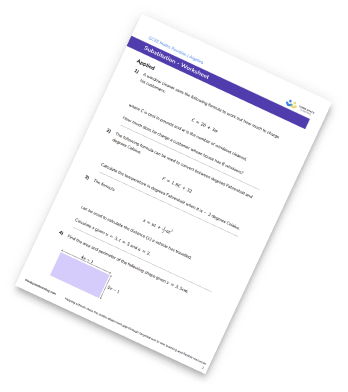Algebraic Fractions Worksheet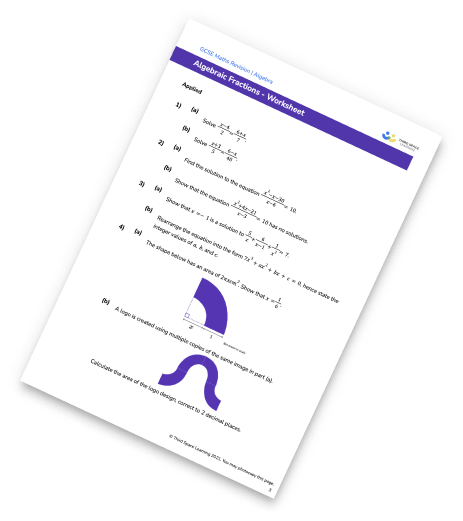Collecting Like Terms Worksheet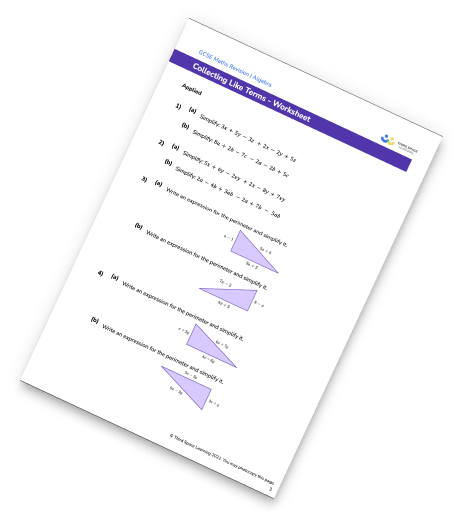Expand and Simplify Worksheet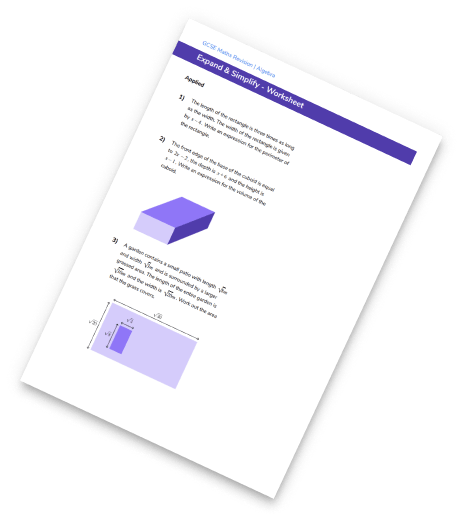## Do you have KS4 students who need more focused attention to succeed at GCSE?There will be students in your class who require individual attention to help them succeed in their maths GCSEs. In a class of 30, it’s not always easy to provide.

Help your students feel confident with exam-style questions and the strategies they’ll need to answer them correctly with our dedicated GCSE maths revision programme.

Lessons are selected to provide support where each student needs it most, and specially-trained GCSE maths tutors adapt the pitch and pace of each lesson. This ensures a personalised revision programme that raises grades and boosts confidence.

Find out more